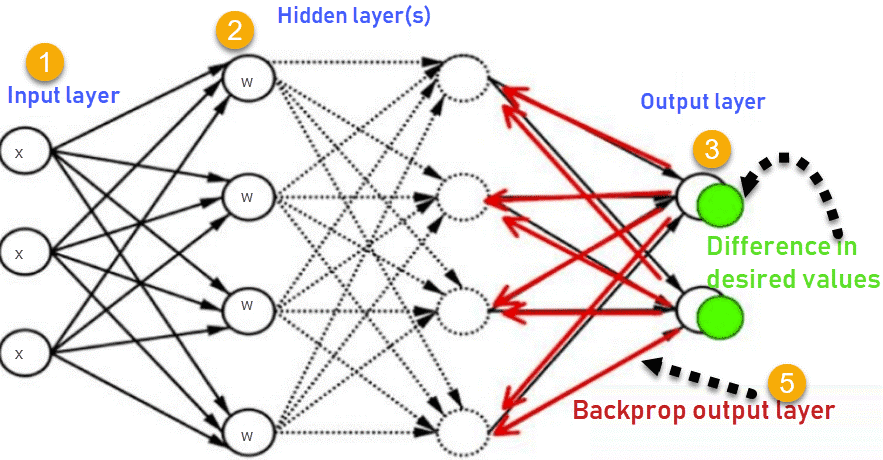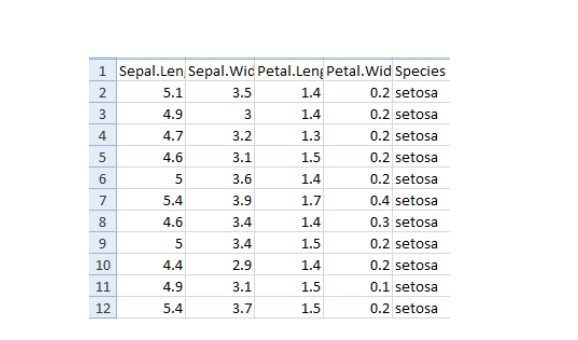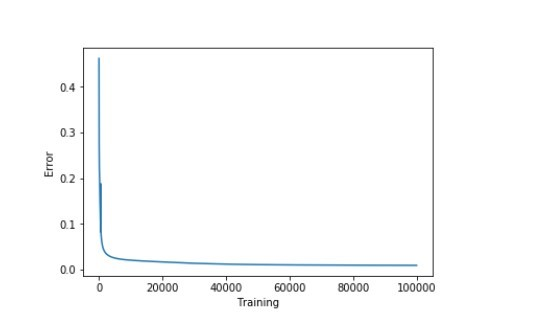# 反向传播算法详解deephub.ai 翻译组

1. 神经网络
2. 什么是反向传播？
3. 反向传播是如何工作的？
4. 损失函数
5. 为什么我们需要反向传播？
6. 前馈网络
7. 反向传播的类型
8. 案例研究

## 反向传播是如何工作的？（How does backpropagation work?）

1.输入层

2.隐藏层

3.输出层1.输入层接收x

2.使用权重w对输入进行建模

3.每个隐藏层计算输出，数据在输出层准备就绪

4.实际输出和期望输出之间的差异称为误差

5.返回隐藏层并调整权重，以便在以后的运行中减少此错误

## 损失函数（Loss function）

•反向传播快速、简单且易于实现

•没有要调整的参数

•不需要网络的先验知识，因此成为一种灵活的方法

•这种方法在大多数情况下都很有效

•模型不需要学习函数的特性

## 反向传播的类型（Types of backpropagation）

•静态反向传播（Static backpropagation）

•循环反向传播（Recurrent backpropagation）

1. 静态反向传播（Static backpropagation）

1. 循环反向传播（Recurrent backpropagation）

## 案例研究（Case Study）

import numpy as np
import pandas as pd
import seaborn as sns
import matplotlib.pyplot as plt
from sklearn.model_selection import train_test_split


import warnings
warnings.simplefilter(action='ignore', category=FutureWarning)


iris = pd.read_csv("iris.csv")iris. replace (, , inplace=True)


1.对输出执行独热编码（one hot encoding）。

2.执行sigmoid函数

3.标准化特征

defto_one_hot(Y):
n_col = np.amax(Y) + 1
binarized = np.zeros((len(Y), n_col))
for i in range(len(Y)):
binarized ] = 1.return binarized


defsigmoid_func(x):return1/(1+np.exp(-x))
defsigmoid_derivative(x):return sigmoid_func(x)*(1 – sigmoid_func(x))


defnormalize(X, axis=-1, order=2):
l2 = np. atleast_1d (np.linalg.norm(X, order, axis))
l2 = 1return X / np.expand_dims(l2, axis)


x = pd.DataFrame(iris, columns=columns)
x = normalize(x.as_matrix())

y = pd.DataFrame(iris, columns=columns)
y = y.as_matrix()
y = y.flatten()
y = to_one_hot(y)


#Split data to training and validation data（将数据拆分为训练和验证数据）
X_train, X_test, y_train, y_test = train_test_split(x, y, test_size=0.33)
#Weights
w0 = 2*np.random.random((4, 5)) - 1#forinput - 4 inputs, 3 outputs
w1 = 2*np.random.random((5, 3)) - 1#for layer 1 - 5 inputs, 3 outputs
#learning rate
n = 0.1


errors = []


for i in range (100000):
#Feed forward network
layer0 = X_train
layer1 = sigmoid_func(np.dot(layer0, w0))
layer2 = sigmoid_func(np.dot(layer1, w1))
layer2_error = y_train - layer2
layer2_delta = layer2_error * sigmoid_derivative(layer2)
layer1_error = layer2_delta.dot (w1.T)
layer1_delta = layer1_error * sigmoid_derivative(layer1)
w1 += layer1.T.dot(layer2_delta) * n
w0 += layer0.T.dot(layer1_delta) * n
error = np.mean(np.abs(layer2_error))
errors.append(error)


accuracy_training = (1 - error) * 100


plt.plot(errors)
plt.xlabel('Training')
plt.ylabel('Error')
plt.show()print ("Training Accuracy of the model " + str (round(accuracy_training,2)) + "%")


Output: Training Accuracy of the model 99.04%

#Validate
layer0 = X_test
layer1 = sigmoid_func(np.dot(layer0, w0))
layer2 = sigmoid_func(np.dot(layer1, w1))
layer2_error = y_test - layer2
error = np.mean(np.abs(layer2_error))
accuracy_validation = (1 - error) * 100print ("Validation Accuracy of the model "+ str(round(accuracy_validation,2)) + "%")


Output: Validation Accuracy 92.86%

•如果约束非常少，则系统可能不起作用

•过度训练，过多的约束会导致过程缓慢

•只关注少数方面会导致偏见

•输入数据是整体性能的关键

•有噪声的数据会导致不准确的结果

•基于矩阵的方法优于小批量方法（mini-batch）04-2337303-208247
04-052533
04-151468
05-095万+
10-30191
04-0115万+
10-03
10-012206
09-153518
10-191万+
01-093万+
11-113万+
11-226155
02-222万+
03-221万+
06-26134
10-272886